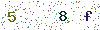﻿ How to understand the intensity distribution curve? - LISUNLISUN

# How to understand the intensity distribution curve?

Definition: Means luminaries or light sources emit light intensity distribution in space. It can record the luminous flux of luminaries, light quantity, power, power factor, the size of the luminaries, luminaries efficiency including manufacturer of luminaries, type and other information. But the most critical is to record the light intensity distribution of luminaries in all directions.

The classification of intensity distribution curve: light distribution curve according to the symmetry properties usually can be divided into: the axial symmetry, symmetric and asymmetric.

1. The axial symmetry: Also known as rotational symmetry, refers to all directions of light distribution curve are basic symmetry, generally, the tube lamp, mining lamp with this intensity distribution.
2. Symmetric: When luminaries C0 ° and C180 ° profile intensity distribution is symmetrical, and meanwhile C90 ° and C270 ° profile light distribution is symmetrical, the light distribution curve is called asymmetric intensity distribution
3. Asymmetric: Means C0 ° and C180 ° and C90 ° and C270 ° an arbitrary profile intensity distribution is not symmetrical.
• Light distribution curve according to the beam Angle is usually divided into: Narrow beam, Wide beam, Secondary beams etc.

Narrow beam: Beam Angle of < 20°

Secondary beam: Beam Angle: 20 ° ~ 40 °

Wide beam: Beam Angle: > 40 °

Any luminaries’ luminous intensity is different in different spatial, we can use the data or graphics to record the luminous intensity in the spatial distribution, usually we use the y coordinate to represent the intensity distribution, centering on the origin of coordinates, and using vector to mark the luminous intensity in different spatial, then connect the endpoint of vector, which form the light intensity distribution curve, this is intensity distribution curve. Since most of the luminaries’ shape is ax symmetric solid of revolution, its luminous intensity distribution in the space is ax symmetric. So, take a point on the luminaries axis of arbitrary surface, use this light intensity distribution curve to represent the intensity distribution of whole space is enough.

If the luminous intensity distribution in space is not symmetrical, for example, linear fluorescent lamps, you need to use a number of light intensity distribution curve in the different surface to illustrate the spatial light distribution. Take the long axis and vertical center surface of lamps as C0 plane , and perpendicular to the CO plane through the center of the lamps as C90 plane. To use C0, C90, at least two plane intensity distribution of lamps is asymmetric. See below:In order to compare the light distribution characteristics of all kinds of lighting lamps , uniform flux for 1000 lumens (lm) imaginary light source to provide intensity distribution data. Therefore, the actual light intensity should be a metering data which provided by the light intensity value multiplied by the ratio of the actual luminous flux and 1000. The lamp’s intensity distribution is the use of the reflectors of lamps, no opaque prism, grating or astigmatism cover control lights to achieve. Reflector is the basic control light parts of lamps, the higher its reflectance, the stronger the regular reflection.